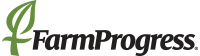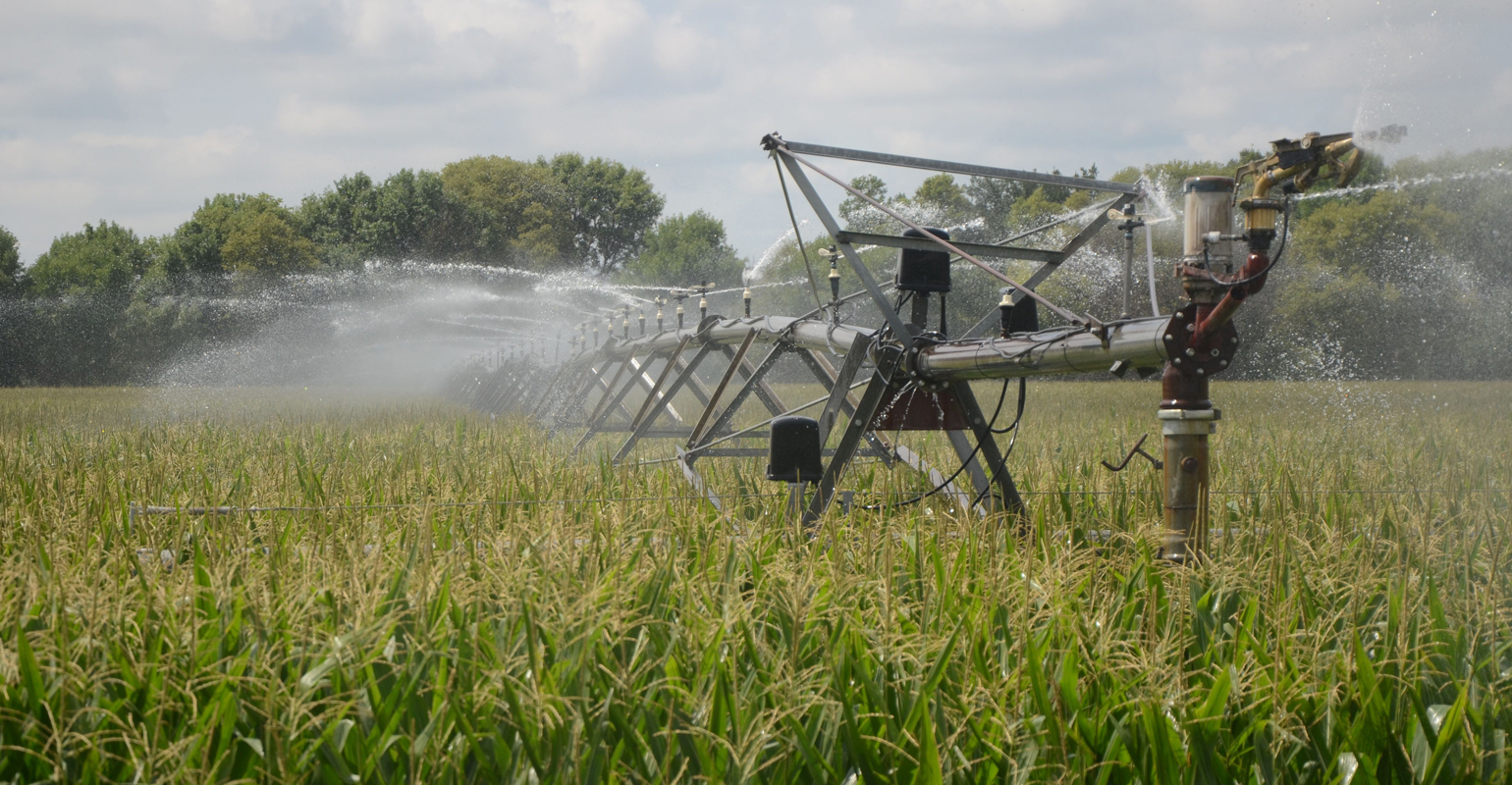# There's more than one way to measure ETThere's more than one way to measure ET

Evapotranspiration is used for decisions regarding irrigation scheduling and prescriptions, but ET is calculated differently in different scenarios.CALCULATING ET: Two of the many ways to calculate ET include indirect and direct measurements. A common indirect method, the two-step approach, relies on a reference ET and a crop coefficient.

When discussing irrigation, evapotranspiration — the amount of water that evaporates and is transpired — is brought up often. It's the biggest user of water in the water balance, accounting for about 80% of the water used. It's used to make irrigation scheduling decisions, and could be used to make variable-rate irrigation prescriptions.

ET can be calculated and measured using dozens of different methods — often expressed in inches per acre, millimeters per acre, or inches or millimeters over a given time frame (inches per day). "There's a lot of confusion based on the number of ways we estimate or model ET," notes Daran Rudnick, Nebraska Extension agriculture water management specialist.

With that in mind, here's a look at some commonly used methods to estimate or measure crop ET, or ETc — indirect and direct methods.

Indirect methods
Indirect methods are often used to estimate crop ET. Two common ones are the two-step crop coefficient approach and the soil-water balance approach:

The two-step crop coefficient approach is the most common equation. It uses a crop coefficient (Kc) and multiplies it by reference ET (ETo): ETc = (Kc) × (ETo).

Reference ET can be calculated several ways. The most common is the Penman Monteith method. Considered the gold standard by the UN's Food and Agriculture Organization, Penman Monteith uses a combination of parameters — radiation, temperature, relative humidity and wind speed — to account for ET.

Another common reference ET method, the Hargreaves equation, relies solely on temperature and may be a viable option when only temperature data is available.

"The Hargreaves equation relies on maximum and minimum temperature and site location. It doesn't factor in solar radiation. Net radiation is a major driver of ET, and temperature is correlated with radiation, but it isn't always that direct," Rudnick says. "All of these factors are tied together and impact how the crop is responding. That's why Penman Monteith works well; it factors in all those things."

With the two-step method, a crop coefficient (Kc) is used to account for all factors affecting the difference in water use between a reference crop (in Nebraska's case, alfalfa) and cash crop. Two methods are used here.

The single coefficient approach uses a single Kc value to account for crop characteristics and averaged effects of soil evaporation, while the dual coefficient approach splits the coefficient into transpiration and evaporation. Transpiration is calculated by multiplying the basal crop coefficient (Kcb) by the water stress coefficient (Ks) and ETo. Evaporation is calculated by multiplying a water evaporative coefficient (Ke) by ETo.

• Soil-water balance (SWB) is another indirect method for calculating ET. True to its name, soil-water balance accounts for the difference between the inputs into the system and the outputs. That is, measuring or modeling precipitation (P), irrigation (I), deep percolation (DP), upward water flux (U), runoff (R) and change in stored soil water (ΔS) over time.

Some parameters, like precipitation, are easier to measure directly. Others have to be estimated using models. For example, runoff is commonly estimated using the Natural Resources Conservation Service’s runoff curve number method — which uses information on land cover, land use and soil type (hydrologic soil group) to determine runoff potential.

These values are plugged into the SWB equation to solve for ETc = P + I + U – R – DP ± ΔS.

Direct methods
Direct methods give an actual measurement of ET. These methods, while not always practical at the farm level, can be used to verify indirect methods. They include eddy covariance and lysimeters:

•Eddy covariance is a technique that measures ET using flux towers like those made by Li-Cor. These flux towers include a sonic anemometer that measures wind speed and direction, and a gas analyzer that measures the concentration of water vapor and carbon dioxide in the air.

Combining the two gives a direct measurement of the "flux" of water vapor and carbon dioxide leaving and returning to the surface of the earth from the atmosphere — giving a direct net measurement of water evaporated and transpired. It relies on air movement across the land surface and works best under turbulent conditions.

Meanwhile, eddy covariance measures latent heat flux — the amount of energy used to evaporate water. It doesn't account for the radiation used to heat plant biomass and the soil, and the radiation reflected. So, additional instrumentation is needed to close the energy balance. Without accounting for total net radiation, some correction is needed to improve the accuracy of the ET measurement.

•Lysimeters are a direct soil-water balance that can be used to measure ET by accounting for the amount of precipitation that falls, and the amount of water that evaporates and transpires. Both weighing and draining lysimeters are installed in the ground (with plants growing in the soil above) and measure the water coming in and leaving.

"It's probably the most direct way to measure ET," Rudnick says. "There's no energy closure; it's just a balanced system of what goes in and what goes out."

But that doesn't mean lysimeters aren't subject to error. They cover a relatively small area, so it's important to place them in a location that's representative of the field. (Some used for USDA research in Texas are about 6.5 by 5 feet.)

"With ET being a primary driver for how we manage irrigation, it's important that we understand the strengths and shortcomings of the various methods used to measure or estimate it," Rudnick says. "Misuse of reliable data, such as using the wrong reference surface crop coefficient, and/or using inaccurate data can result in over- or under-irrigation, and ultimately, negatively affect operational expenses and farm profitability."